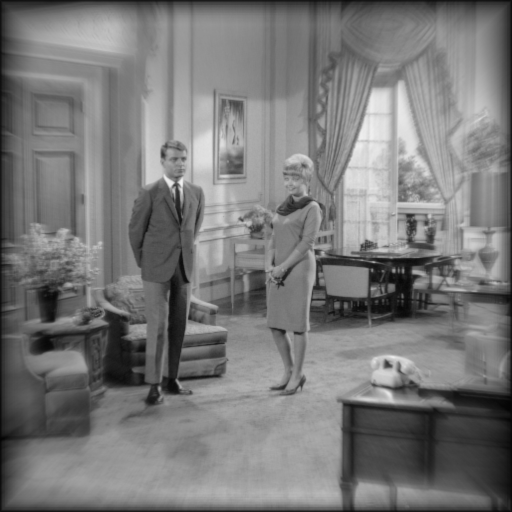# Seidel Aberration Imaging

## Introduction

The purpose of this page is to demonstrate how the Seidel Aberrations affect image quality. Unlike the other parts of this website, this is an offline/noninteractive demonstration due to the immense processing power required to perform each simulation.

## Method

The Seidel Aberrations are specified using three subscripts, where each subscript is the power of each of the wavefront power series expansion terms: $$W_{ijk} = H^{i}\rho ^{j}\cos ^{k}\theta$$ The image is generated using the superposition integral $$I(x,y) = \iint_{-\infty }^{\infty }G(x,y)\left | \Im^-1 (P(-\lambda zf_U, -\lambda z f_V)) \right |^2dxdy$$ where $P(-\lambda zf_U, -\lambda z f_V)$ is the pupil function $$P(x_p, y_p) = circ\left ( \frac{\sqrt{x_p^2 + y_p^2}}{r_{xp}} \right )e^{-ikW(x_p,y_p)}$$ and $G(x,y)$ is the ideal nonaberrated image.

A simulation was performed for each of the first, third, and fifth order Seidel Aberrations. Each simulation contains three waves of the specified aberration. Simulations were performed on two images: the 1951 USAF Resolution Chart and the "Living Room" scene (a test image widely used throughout the history of image science).

## Optical System Parameters

For this offline demonstration, the following optical system parameters were specified:
Parameter Value
Image plane side length 1 $mm$
Wavelength 500 $nm$
Exit pupil diameter 20 $mm$
Exit pupil to image distance 100 $mm$
FFT Size 512x512

References:
1. Computational Fourier Optics by David Voelz, SPIE Press, 2011
2. Numerical Simulation of Optical Wave Propagation with Examples in MATLAB by Jason D. Schmidt, SPIE Press, 2010

## Results

#### Image Field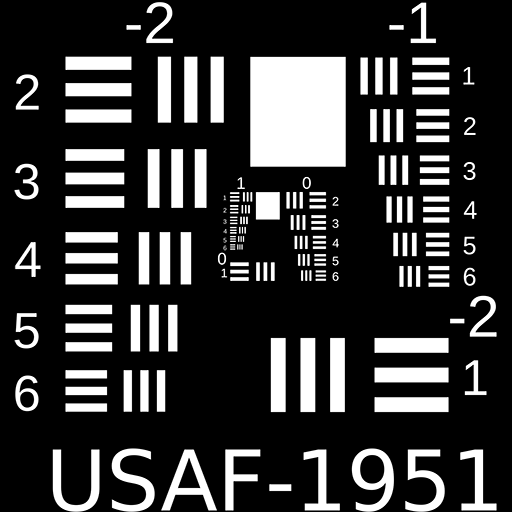#### Diffraction Limited Image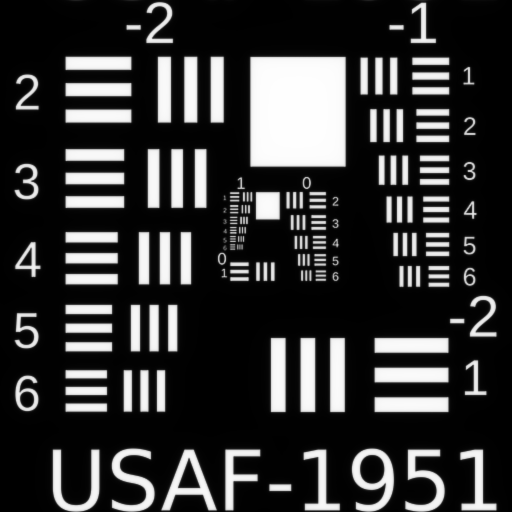#### First Order Defocus $W_{020}$#### First Order Tilt $W_{111}$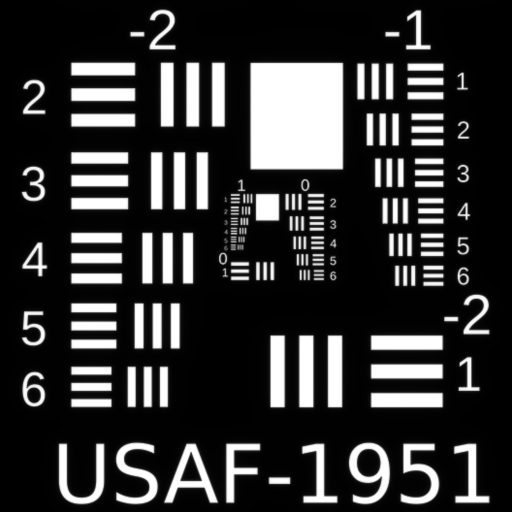#### Third Order Spherical $W_{040}$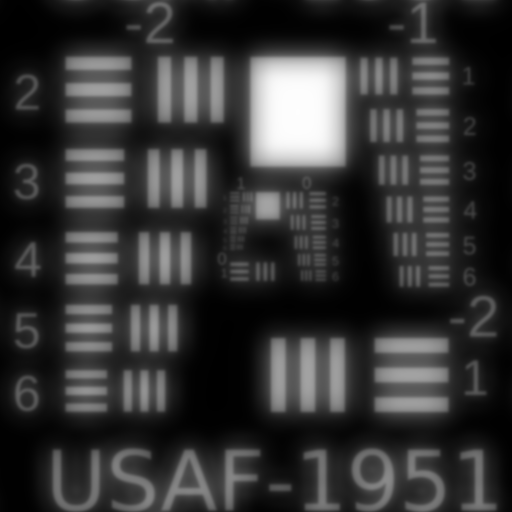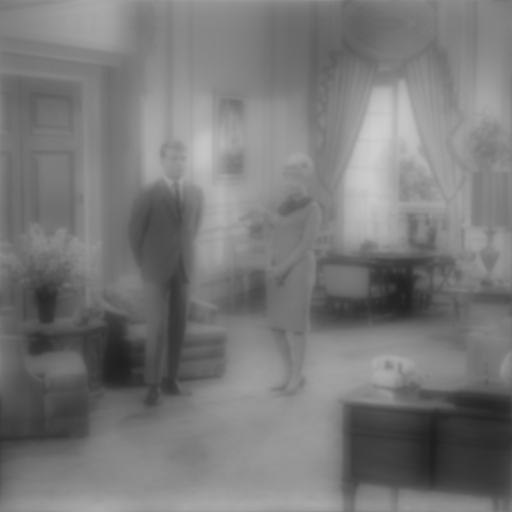#### Third Order Coma $W_{131}$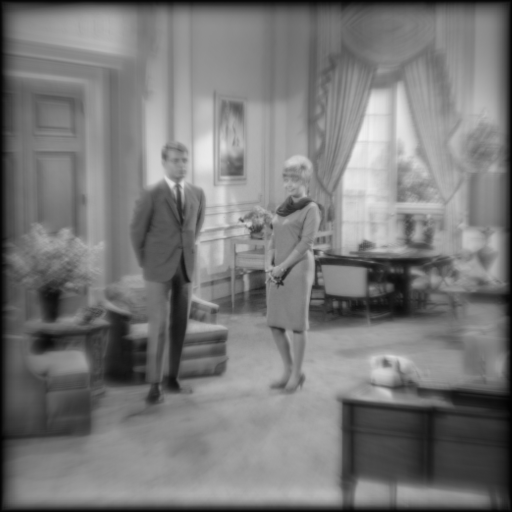#### Third Order Astigmatism $W_{222}$#### Third Order Field Curvature $W_{220}$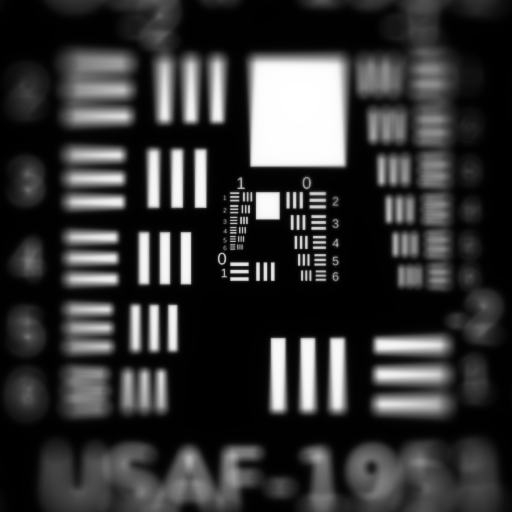#### Third Order Distortion $W_{311}$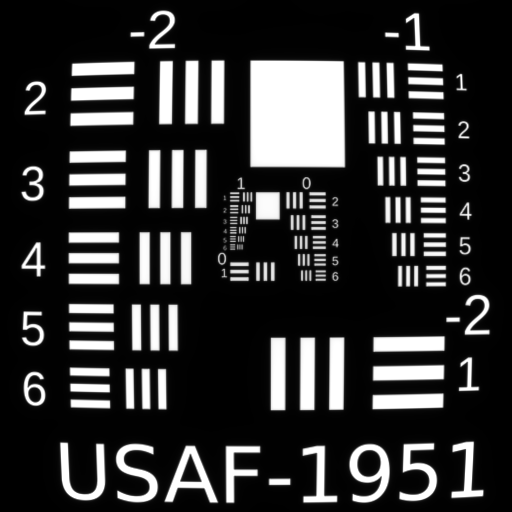#### Fifth Order Spherical $W_{060}$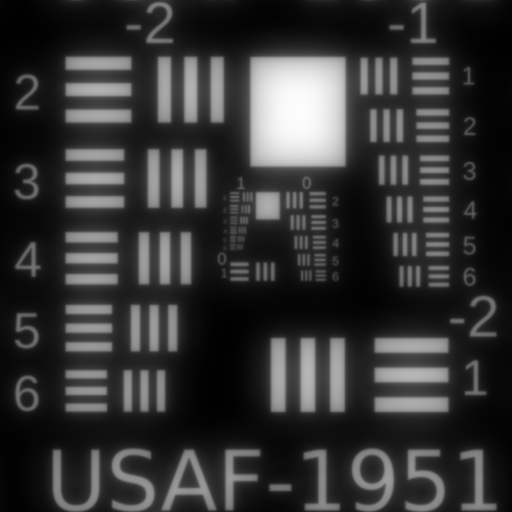#### Fifth Order Sagittal Oblique Spherical $W_{240}$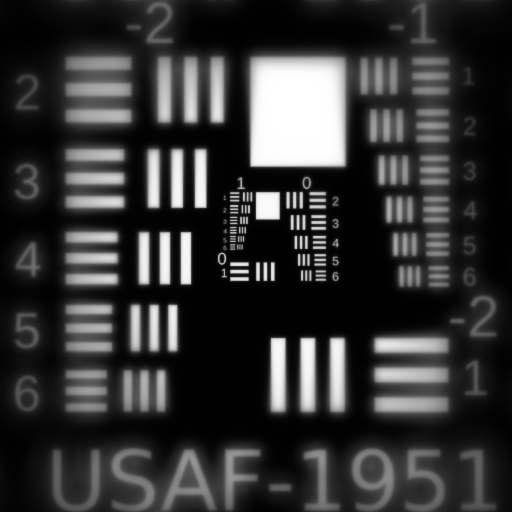#### Fifth Order Field Curvature $W_{420}$#### Fifth Order Linear Coma $W_{151}$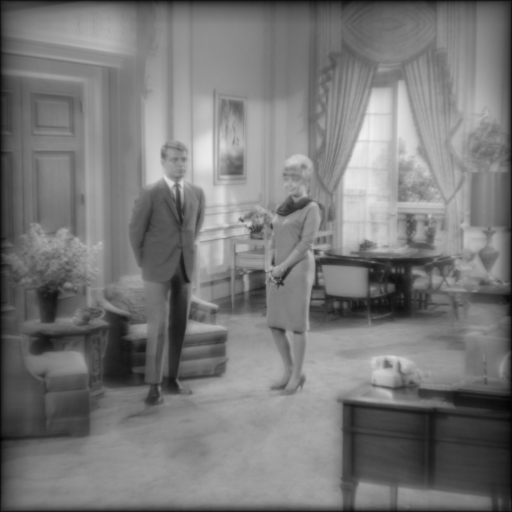#### Fifth Order Cubic Coma $W_{331}$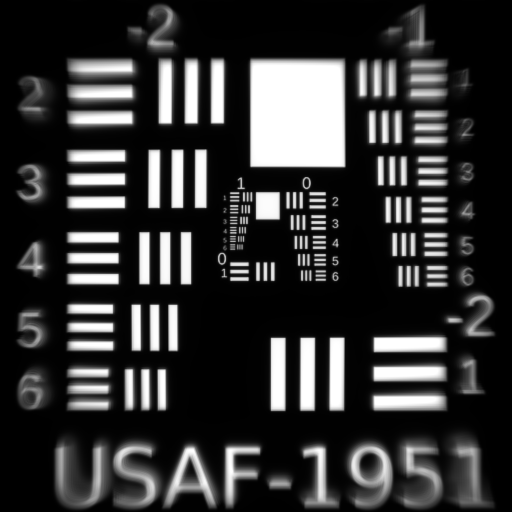#### Fifth Order Distortion $W_{511}$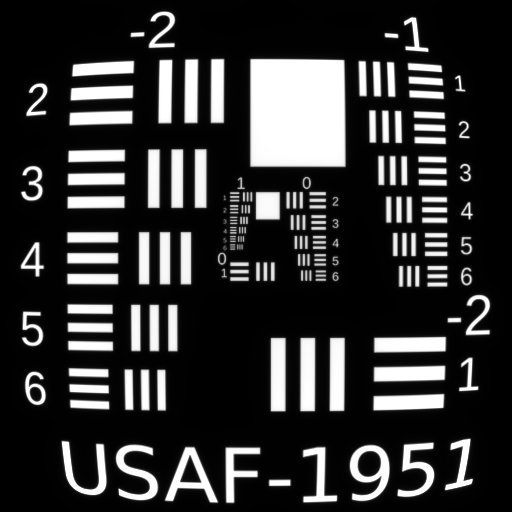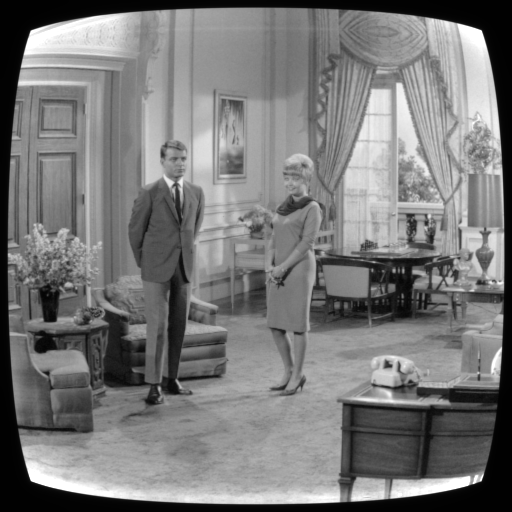#### Fifth Order Tangential Oblique Spherical $W_{242}$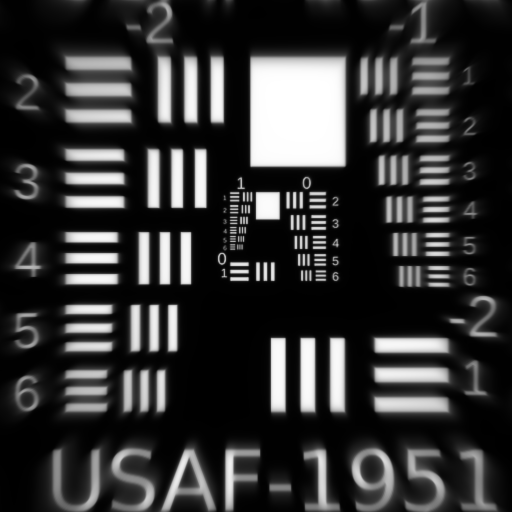#### Fifth Order Astigmatism $W_{422}$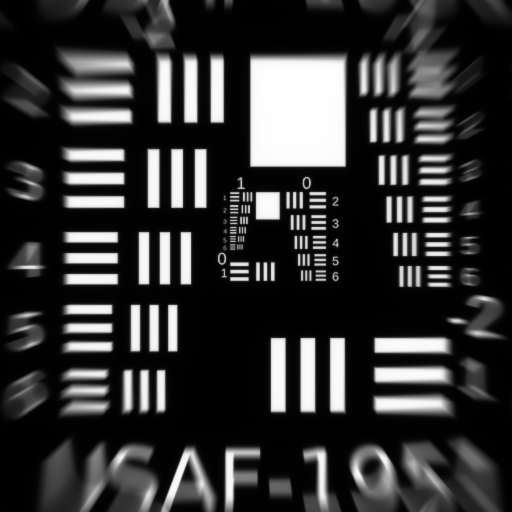#### Fifth Order Line-Elliptical Coma $W_{333}$